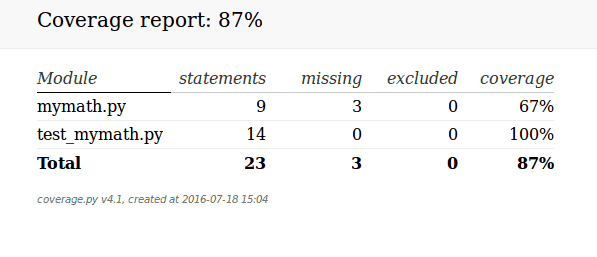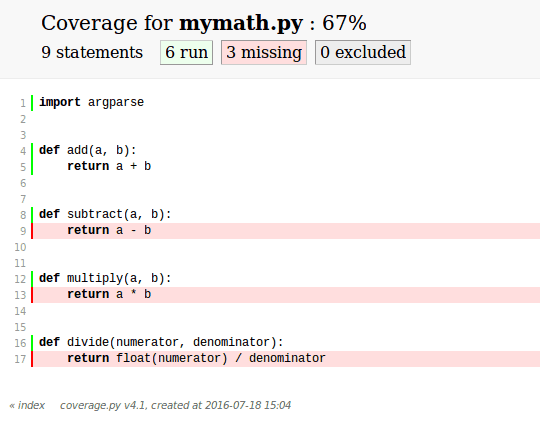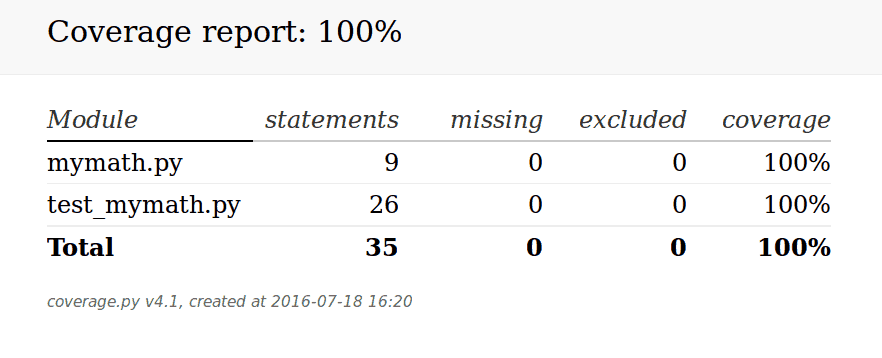Coverage.py 是一个用来测试代码覆盖率的 Python 第三方库。它起初是由 Ned Batchelder 创建。在编程界，术语“覆盖”通常是用来描述测试的有效性，以及测试的实际覆盖率。coverage.py 库支持 Python 2.6 或者更高的版本，还兼容 Python 3 的最新版以及 PyPy 。

```pip install coverage
```

```def add(a, b):
return a + b

def subtract(a, b):
return a - b

def multiply(a, b):
return a * b

def divide(numerator, denominator):
return float(numerator) / denominator
```

```# test_mymath.py
import mymath
import unittest

"""
Test the add function from the mymath library
"""

"""
Test that the addition of two integers returns the correct total
"""
self.assertEqual(result, 3)

"""
Test that the addition of two floats returns the correct result
"""
self.assertEqual(result, 12.5)

"""
Test the addition of two strings returns the two string as one
concatenated string
"""
self.assertEqual(result, 'abcdef')

if __name__ == '__main__':
unittest.main()
```

```coverage run test_mymath.py
```

```coverage report -m
```

```Name             Stmts   Miss  Cover   Missing
----------------------------------------------
mymath.py            9      3    67%   9, 13, 17
test_mymath.py      14      0   100%
----------------------------------------------
TOTAL
```

-m 选项告诉 coverage.py 你想在输出信息中显示 Missing 列。如果省略 -m 选项，就只能看到前四列信息。上面的输出表明，coverage 在执行完测试代码之后，判断我写的单体测试程序对 mymath 模块的覆盖率只有 67% 。 “Missing” 列表明哪些行代码没有被覆盖。如果你看过 coverage.py 指出的那些行代码，很快就会发现测试程序没有运行测试 subtract, multiplydivide 函数。

```coverage html
``````class TestSubtract(unittest.TestCase):
"""
Test the subtract function from the mymath library
"""

def test_subtract_integers(self):
"""
Test that subtracting integers returns the correct result
"""
result = mymath.subtract(10, 8)
self.assertEqual(result, 2)
``````class TestMultiply(unittest.TestCase):
"""
Test the multiply function from the mymath library
"""

def test_subtract_integers(self):
"""
Test that multiplying integers returns the correct result
"""
result = mymath.multiply(5, 50)
self.assertEqual(result, 250)

class TestDivide(unittest.TestCase):
"""
Test the divide function from the mymath library
"""

def test_divide_by_zero(self):
"""
Test that multiplying integers returns the correct result
"""
with self.assertRaises(ZeroDivisionError):
result = mymath.divide(8, 0)
```额外信息

Coverage.py 也允许在上述提到的配置文件中指定你需要解析的源文件。一旦在配置文件中设置了需要解析的文件，就可以通过运行 coverage.py 来看运行结果。它还支持“-source”命令行选项。最后，还可以使用“-include”和“-omit”选项来包含或者移除一个文件名模式的列表。这些选项也可以通过在配置文件中添加对应的配置项进行设置。

总结

Python 翻译组是EarlGrey@编程派发起成立的一个专注于 Python 技术内容翻译的小组，目前已有近 30 名 Python 技术爱好者加入。